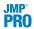Suppose you want to test whether the mean profit earned by companies differs by type of company. In Companies.jmp, the data consist of various metrics on two types of companies, Pharmaceutical (12 companies) and Computer (20 companies).
 1 Select Help > Sample Data Library and open Companies.jmp.
 2 Select Analyze > Fit Y by X.
 3 Select Profits (\$M) and click Y, Response.
 4 Select Type and click X, Factor.
 5 Click OK.
 6 From the red triangle menu, select Display Options > Box Plots.
Computer Company Profit Distribution
 7 From the red triangle menu, select Means/ANOVA/Pooled t.
Company Analysis of Variance
The F test shows no significance because the p-value is large (p = 0.1163). This might be due to the large value in row 32 and the possible violation of the normality assumption.
 8
t-Test Results
 9 From the red triangle menu, select Nonparametric > Wilcoxon Test.
Wilcoxon Test Results
Both the normal and the chi-square approximations for the Wilcoxon test statistic indicate significance at a p-value of 0.0010. You conclude that there is a significant difference in the location of the distributions, and conclude that mean profit differs based on company type.
 10From the red triangle menu, select Nonparametric > Exact Test > Wilcoxon Exact Test.
Wilcoxon Exact Test Results
The observed value of the test statistic is S = 283. This is the sum of the midranks for the level of Type with the smaller sample size (pharmaceuticals). The probability of observing an absolute difference from the mean midrank that exceeds the absolute value of S minus the mean of the midranks is 0.0005. This is a two-sided test for a difference in location and supports rejecting the hypothesis that profits do not differ by type of company.
In this example, the nonparametric tests are more appropriate than the normality-based ANOVA test and the unequal variances t-test. The nonparametric tests are resistant to the large value in row 32 and do not require the underlying normality of Profits (\$M) for each group.

Help created on 9/19/2017